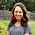## Monday, July 23, 2012

### More thoughts on the Chain Rule

I posted about how teaching the Chain Rule was a lot harder than I thought it would be.  I'm still convinced this is at least partially due my students' lack of understanding/recognizing a composite function.  For example, just the other day we needed to simplify the expression

And a student (one of my top students, I might add) suggested we "divide out an ln."

Hold up.

Let's ignore the fact that dividing by any number other than 1 would change the expression.

Dividing by ln?  So...somehow there's not a connection that ln is meaningless without an argument.  "Dividing by ln" is akin to "dividing by √ " or "dividing by cos."  An empty square root or an empty cosine doesn't have any kind of value, and really doesn't mean a thing.

I was further disturbed when I gave my Business Calculus students a function like

and was told that in order to find the derivative, we should use the Product Rule.

Wha...?

Do students view ln as some sort of constant?  Like e?  It seems maybe so if the function above is thought of as a product and if we can indeed "divide out ln."

I decided we needed to revisit the Chain Rule.

I started by showing a slide that had a composite function at the top and four expressions beneath it such as:

I asked my students to tell me why we needed to use the Chain Rule and then to identify the derivative of the outside function (holding the inside) and the derivative of the inside function.  The next slide highlighted the former in red and the latter in blue:

We did several of these.  Then we concluded with other types of functions to test if they knew when to use what rules.

I think I will start with this type of presentation the next time I teach the Chain Rule.  Giving the students a limited amount of options to start out with seemed to worked fairly nicely.

There are still definitely some issues.  But I now have a better understanding of what needs to be emphasized  in terms of composite functions.  I will try to make them more densely populated in my algebra and pre-calculus classes from now on.

If you're interested, here's the slideshow we worked through:

Test 3 Review

1.My guess is their problems were more with the logarithm function than with chain rule. So I'd review logs too.

For chain rule, I try to get them to use the word something as much as possible to see the structure of an expression:
log of something
something times something
something to the something power
square root of something

And I talk about the chain rule helping them to peel off the outer layer first, like an onion.

2.Hay dude yes the chain rule very difficult, thanks for the share. Product Proposals

3.Sue--

Oooo! I like the onion analogy! Stealing it. :)

I thought it was a log issue as well, until I got similar responses for trig functions, too. For example, we had an expression such as x+cos(2x), and a student asked why we can't factor out an x (and this wasn't an isolated comment). It was comments like these that made me think a lot of students don't just "see" the inside function. What's been your experience with composite functions?

Also, when I was helping a student one-on-one with derivatives of log functions, he made the comment, "But my College Algebra teacher told me ln is just a number." Which, surely didn't happen, but really got me thinking...it seems like there's some kind of disconnect for compositions that isn't there for the other 4 combinations of functions.

4.You may be right, but I'd blame it more on not understanding functions themselves, rather than on not understanding composition. A way to test my hypothesis would be to ask them to give the derivative of the square root of (x^2+1). They've dealt with square roots for much longer than they've dealt with trig or logs. Plus, it doesn't have the parentheses that make them think 'multiplication' (!). OK, I have to remember this, I'm going to quiz mine in the fall on 3 functions: a square root, a trig function, and a log. Each will have a simple function of x inside.

We know that teacher said, "e is just a number". I say it all the time. I never imagined a student would/could misremember it this way!

1.Exactly! They seem so comfortable with the square root function. I don't know why I didn't think of this before, but maybe giving them f(x)=sqrt(x^2+1), f(x)=sin(x^2+1), and f(x)=ln(x^2+1) all in a row will help. Keep the inside functions the same for the first example. Thanks, Sue!!!

5.Hey I had very similar problems with my students. The last thing you pointed out in your comments was the thing that really helped. Some still had trouble with e to a function though. One thing that helped with that was asking them to plug in 5 or some other random number (by hand, not with the calculator). Then they can see what they do... Oh you do the stuff in the exponent first, then take e to the whole thing. So e has to be the noutside function, or the second function.

6.Also you can probably tell I'm working through my backed up google reader :)

1.Oooo I like plugging in a number for the ind. variable. That's something concrete I can use when students are struggling with identifying the inside and outside functions. Brilliant!

Tell me what you think!